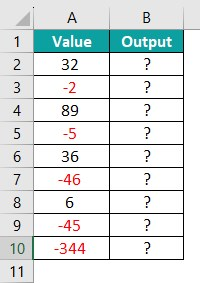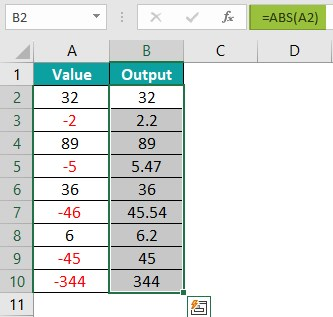# Excel Negative NumbersArticle byExcelMojo Team## What Are Negative Numbers in Excel?

The Excel Negative Numbers are those lower than zero on the number scale. Those are the ones which has a minus symbol as a prefix in Excel. This is easy and simple in Excel to use with negative numbers, but it can create confusion for us due to the different visibility of the same. The simple rule is that any number with a minus sign as a prefix is a negative number.

There are various states of showing or highlighting negative numbers, those numbers are with sales, margin, scores, and financial data now and then, and we can deal.

For example, the image below expects the Value in column A. We will apply for the Negative Numbers in Excel to evaluate the negative value as the output.

The entered in cell A2; is returned as ‘-2,’ as shown below.

###### Key Takeaways
• Excel handles positive and negative numbers effectively; The issue while handling negative numbers is the use of inappropriate format.
• The Conditional formatting technique is used to highlight negative numbers.
• The ABS function is used to convert negative numbers into positive numbers.

### Explanation

The Negative Numbers with a “-” minus sign are very easy to analyze. Still, when the mix of positive and negative values is presented, it needs to change the format of the Excel cells that contain negative values to get accurate results.

To edit negative values in an Excel sheet, we can help the working with negative data points and values. The reasons for using Negative Numbers in Excel include step-by-step instructions for two methods for making excel negative numbers.

### Top 3 Ways To Show / Highlight Negative Numbers In Excel

1. Conditional Formatting – Conditional formatting highlights certain values or makes particular cells easy to identify. This highlights the cells that contain values that meet a certain condition.
2. Inbuilt Number Formatting- The number formatting is used to change the appearance of numbers, including dates and times, without changing the actual number. The actual value is displayed in the formula bar.
3. Custom Number Formatting- The Custom Number Formatting changes only the visual representation of the value in a cell. Remember, we cannot change or delete the original number format.

#### #1 – Example With Conditional Formatting

The succeeding example depicts the values, and we will show or highlight the Negative Numbers of the given values using Conditional Formatting.

In the table,

• Column A contains the Value.

The steps to show or highlight the value by the Conditional Formatting are as follows:

Step 1: Select the cell with the values. In this case, cell A2:A10.

Step 2:  Select the Conditional Formatting option from the Style group of the Home tab.

Step 3:  Select Highlight Cells Rules from the drop-down list.

Step 4:  Select Less Than… from the drop-right list.

Step 5:  The window called Less Than pops up.

Step 6:  Enter the value in the Format cells that are LESS THAN: box and select Light Red Fill with Dark Red Text from the list to highlight.

Step 7:  Select OK.

Step 8:  The cells less than -20 cells are highlighted with Light Red filled with Dark Red Text.

#### #2 – Example With Inbuilt Number Formatting

The succeeding example depicts the values, and we will show or highlight the Negative Numbers of the given values using the Inbuilt Number Formatting.

In the table,

• Column A & B contains the Value.

The steps to show or highlight the value by the Inbuilt Number Formatting are as follows:

Step 1: Select the cell with the values. In this case, it is cell range A2:B10.

Step 2: Select the arrow button of the Number group of the Home tab. The Number Format checks out the full set of number formatting options.

Step 3: The window called the Format Cells pops up.

Step 4: Select Number from the category list of the Number menu.

Step 5: Select the Negative Number Format.

Step 6: Click OK.

Step 7: All the negative numbers are highlighted in red with the minus sign, as shown in the below image.

#### #3 – Example With Custom Number Formatting

The succeeding example depicts the values, and we will show or highlight the Negative Numbers of the given values using the Custom Number Formatting.

In the table,

• Column A s& B contains the Value.

The steps to show or highlight the value by the Custom Number Formatting are as follows:

Step 1: Select the cell where we have to enter the values. In this case, cell A2:B10.

Step 2: Select the arrow button of the Number group of the Home tab. The Number Format checks out the full set of number formatting options.

Step 3: The window called the Format Cells pops up.

Step 4: Select Custom from the category list of the Number menu.

Step 5: Select the Negative Number Format we wish for or form our format.

Step 6: Click OK.

Step 7: We can see all the negative numbers are highlighted in red color within parentheses.

### Important Things to Note

• The Conditional formatting is a volatile feature; values change in the Excel sheet, and it reassesses the condition and changes the formatting of the dataset. The large datasets might face problems.
• We can use different colors to highlight Negative Numbers in Excel cells.

1. What are the reasons for making Numbers Negative in Excel?

The two reasons for negative numbers in excel are:
Calculating a Loss- Enter the data and formulas to calculate a loss or decrease in a report using negative values.
Presenting Budget Report- When the budget report is formed or created, we use negative values to show areas with negative budgets and areas of expense.

2. How to change Negative numbers to Positive Numbers?

Consider the below table showing value and output in columns A and B, respectively.The steps to calculate the value by the ABS Excel Function are:

Step 1: First, select cell B2 and enter the ABS Excel Function formula =ABS(A2).
Step 4: Press the Enter key. We can see the result in cell B2.

Step 6: Press Enter and drag the cursor to cell B10, as shown in the following image.Note: We can see all the negative numbers are converted into positive ones.

3. How to make Excel Numbers Negative using multiplication formulas?

To make Excel Numbers Negative using multiplication formulas,

Identify the data – Detect the cell position of the data or data range become negative. The cell position allows us to write the necessary formula correctly.
Create a formula to multiply by ‘-1’- Using the correct cell location, a formula multiplies the cell by a negative one. The formula looks like this: =A2 * -1.
Apply for all Desired Results – Apply the formula to the remaining values using the cursor. Select the cell with the original formula and place the cursor at the bottom right corner. Use this symbol cursor to highlight the data range by clicking and dragging it across the cells. This applies the formula to the selected cells and makes all the data.